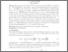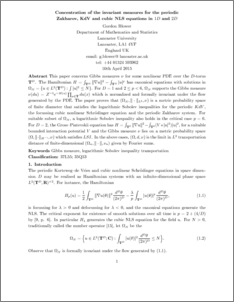# Concentration of the invariant measure for the periodic Zakharov, KdV and cubic NLS equations in 1D and 2D

Blower, Gordon (2016) Concentration of the invariant measure for the periodic Zakharov, KdV and cubic NLS equations in 1D and 2D. Journal of Mathematical Analysis and Applications, 438 (1). pp. 240-266. ISSN 0022-247XPreview
PDF (sturm3)
sturm3.pdf - Accepted Version

## Abstract

This paper concerns Gibbs measures ν for some nonlinear PDE over the D -torus TD. The Hamiltonian H=∫TD‖∇u‖2−∫TD|u|p has canonical equations with solutions in ΩN={u∈L2(TD):∫|u|2≤N}; this N is a parameter in quantum field theory analogous to the number of particles in a classical system. For D=1 and 2≤p<6, ΩN supports the Gibbs measure ν(du)=Z−1e−H(u)∏x∈Tdu(x) which is normalized and formally invariant under the flow generated by the PDE. The paper proves that (ΩN,‖⋅‖L2,ν) is a metric probability space of finite diameter that satisfies the logarithmic Sobolev inequalities for the periodic KdV , the focussing cubic nonlinear Schrödinger equation and the periodic Zakharov system. For suitable subset of ΩN, a logarithmic Sobolev inequality also holds in the critical case p=6. For D=2, the Gross–Piatevskii equation has H=∫T2‖∇u‖2−∫T2(V⁎|u|2)|u|2, for a suitable bounded interaction potential V and the Gibbs measure ν lies on a metric probability space (Ω,‖⋅‖H−s,ν) which satisfies LSI . In the above cases, (Ω,d,ν) is the limit in L2 transportation distance of finite-dimensional (Ωn,‖⋅‖,νn) given by Fourier sums.

Item Type:
Journal Article
Journal or Publication Title:
Journal of Mathematical Analysis and Applications
This is the author’s version of a work that was accepted for publication in Journal of Mathematical Analysis and Applications. Changes resulting from the publishing process, such as peer review, editing, corrections, structural formatting, and other quality control mechanisms may not be reflected in this document. Changes may have been made to this work since it was submitted for publication. A definitive version was subsequently published in Journal of Mathematical Analysis and Applications, 438, 1, 2016 DOI: 10.1016/j.jmaa.2016.01.080
Uncontrolled Keywords:
/dk/atira/pure/subjectarea/asjc/2600/2604
Subjects:
Departments:
ID Code:
78185
Deposited By:
Deposited On:
10 Feb 2016 08:56
Refereed?:
Yes
Published?:
Published